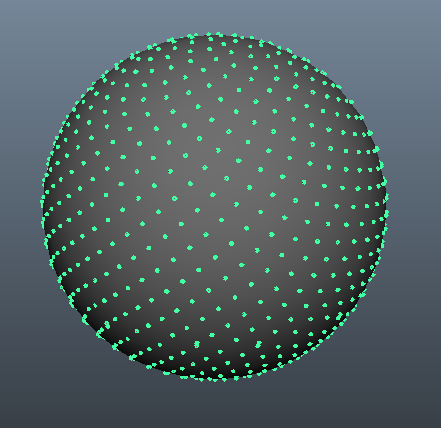# #StackBounty: #math #pseudocode Evenly distributing points on the surface of hyperspheres in higher dimensions

### Bounty: 250

I am interested in evenly distributing N points on the surface of spheres in dimensions 3 and higher.

To be more specific:

• Given a number of points N and number of dimensions D (where D > 1, N > 1)
• The distance of every point to the origin must be 1
• The minimum distance between any two points should be as large as possible
• The distance of each point to it’s closest neighbour doesn’t necessarily have to be the same for every point (indeed it’s not possible for it to be the same unless the number of points forms the vertices of a platonic solid or if N <= D).

I am not interested in:

• Creating a uniform random distribution on a hypersphere, because I want the minimum distance between any two points to be as large as possible instead of being randomly distributed.
• Particle repulsion simulation type methods, because they are hard to implement and take an extremely long time to run for large N (Ideally the method should be deterministic and in O(n)).

One method that satisfies these criteria is called the fibonacci lattice, but I have only been able to find code implementations for that in 2d and 3d.

The method behind the fibonacci lattice (also known as the fibonacci spiral) is to generate a 1d line that spirals around the surface of the sphere such that the surface area covered by the line is roughly the same at every turn. You can then drop N points equally distributed on the spiral and they will roughly be evenly distributed on the surface of the sphere.

In this answer there is a python implementation for 3 dimensions that generates the following:I wanted to know whether the fibonacci spiral could be extended to dimensions higher than 3 and posted a question on the maths stack exchange. To my surprise I received two amazing answers which as far as I can tell (because I don’t fully understand the maths shown) shows it’s indeed possible to extend this method to N dimensions.

Unfortunately I don’t understand enough of the maths shown to be able to turn either answer into (pseudo)code. I am an experienced computer programmer, but my maths background only goes so far.

I will copy in what I believe to be the most important part of one of the answers below (unfortunately SO doesn’t support mathjax so I had to copy as an image)Difficulties presented by the above that I struggle with:

• How to resolve the inverse function used for ψn?
• The example given is for d = 3. How do I generate formulae for arbitrary d?

Would anyone here who understands the maths involved be able to make progress towards a pseudo code implementation of either answer? I understand a full implementation may be quite difficult so I’d be happy with a part implementation that leads me far enough to be able to complete the rest myself.

To make it easier, I’ve already coded a function that takes spherical coordinates in N dimensions and turns them into cartesian coordinates, so the implementation can output either one as I can easily convert.

Additionally I see that one answer uses the next prime number for each additional dimension. I can easily code a function that outputs each successive prime, so you can assume that’s already implemented.

Failing an implementation of the fibonacci lattice in N dimensions. I’d be happy to accept a different method that satisfies the above constraints.

Get this bounty!!!

This site uses Akismet to reduce spam. Learn how your comment data is processed.